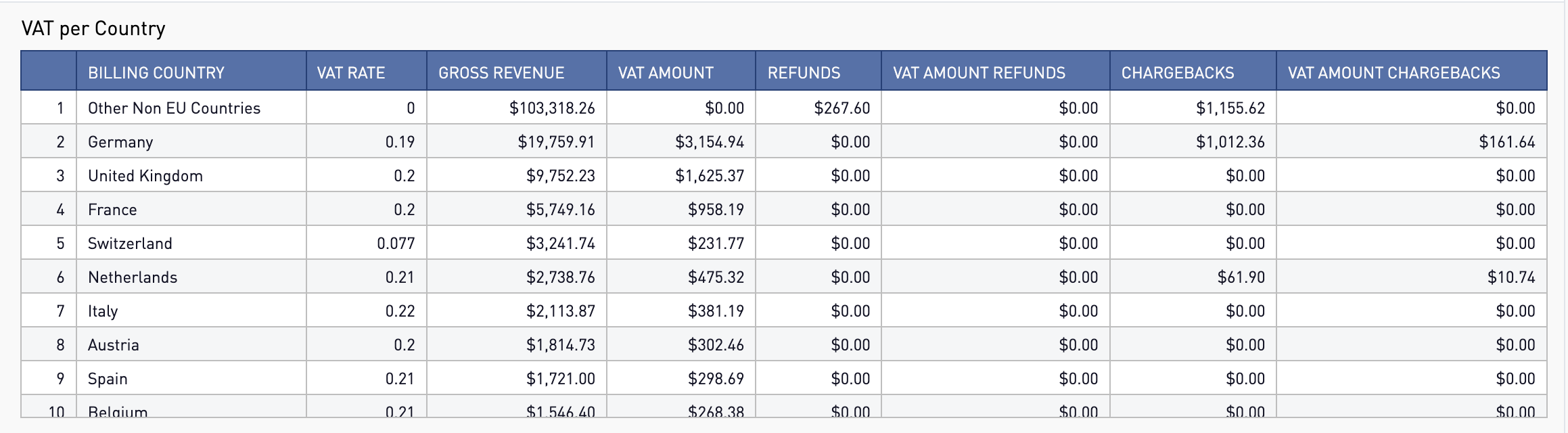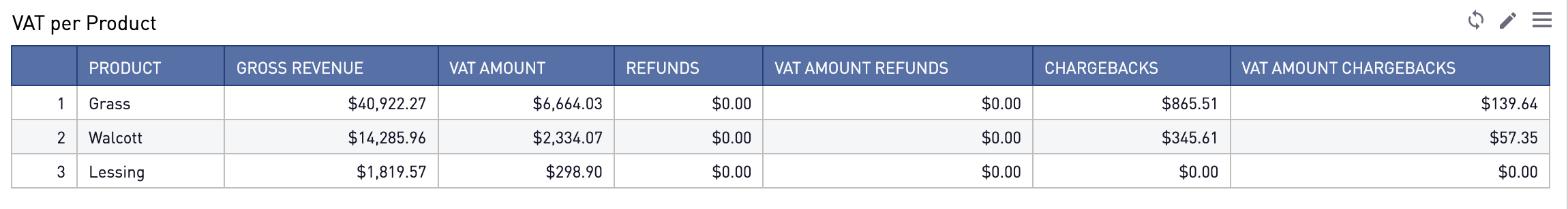# VAT Report

## VAT per country

How much VAT do I need to pay for each country?## VAT per product

How much VAT do I need to pay for each product?# The Terms

VAT Rate

VAT Rate of each country in Europe.

VAT is collected on behalf of governments from consumers. This is very common in Europe. It is similar to a sales tax or consumption tax in the United States.

# The Math

Gross Revenue

It is the total revenue for all transaction types excluding chargebacks, refunds and partial refunds.

VAT Amount

VAT amount = ((Gross Revenue) / (1 + VAT Rate)) * (VAT Rate) = ((20/(1 + 0.19)) x 0.19

Refunds

The total amount of refunds and partial refunds in a period.

VAT Amount Refunds

VAT amount refunds = ((Refunds)/(1+ VAT Rate)) * (VAT Rate)

Chargebacks

The total amount of Chargebacks in a period.

VAT Amount Chargebacks

VAT amount chargebacks = ((Chargebacks)/(1+VAT Rate)) * (VAT Rate)